Chin. Phys. B, 2020, Vol. 29(10): 106301    DOI: 10.1088/1674-1056/abad21
 CONDENSED MATTER: STRUCTURAL, MECHANICAL, AND THERMAL PROPERTIES Prev   Next

# Acoustic topological phase transition induced by band inversion of high-order compound modes and robust pseudospin-dependent transport

Yan Li(李妍)1,†(), Yi-Nuo Liu(刘一诺)1, Xia Zhang(张霞)1
1 School of Physics and Physical Engineering, Qufu Normal University, Qufu 273165, China
Abstract

A simple two-dimensional phononic crystal hosting topologically protected edge states is proposed to emulate the quantum spin Hall effect in electronic systems, whose phononic topological phase can be reconfigured through the rotation of scatters. In particular, the band inversion occurs between two pairs of high-order compound states, resulting in topological phase transition from trivial to nontrivial over a relatively broad high-frequency range. This is further evidenced by an effective Hamiltonian derived by the kp perturbation theory. The phononic topology is related to a pseudo-time-reversal symmetry constructed by the point group symmetry of two doubly degenerate eigenstates. Numerical simulations unambiguously demonstrate robust helical edge states whose pseudospin indices are locked to the propagation direction along the interface between topologically trivial and nontrivial phononic crystals. Our designed phononic systems provide potential applications in robust acoustic signal transport along any desired path over a high-frequency range.

Keywords:  topological phase transition      band inversion      compound mode      pseudospin
Received:  02 July 2020      Revised:  30 July 2020      Published:  05 October 2020
 PACS: 63.20.-e (Phonons in crystal lattices) 43.35.Gk (Phonons in crystal lattices, quantum acoustics) 43.40.+s (Structural acoustics and vibration) 46.40.-f (Vibrations and mechanical waves)
Corresponding Authors:  Corresponding author. E-mail: liyanQFNU@163.com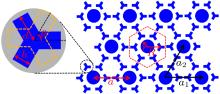Fig. 1.  Schematic view of a triangular phononic crystal consisting of snowflake-like metamolecules. Red dashed hexagon indicates the primitive cell composed by one cylindrical iron rod and six three-legged iron rods embedded in an air host. a is the lattice constant. a1 and a2 are the unit vectors. R = 0.27a denotes the distance between the centers of cylindrical rods to the centers of three-legged rods. The radii of the center cylindrical rods are r = 0.1a. The width and length of the legs are h = 0.03a and d = 0.135a, respectively. The angle θ with respect to the horizontal axis characterizes the orientation of the three-legged iron rods.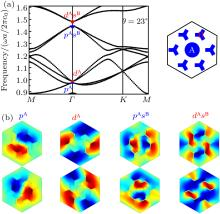Fig. 2.  (a) Band structure for the triangular phononic crystal with rotational angle θ = 23°. The right inset shows the primitive cell diagram. The cylindrical rod and three-legged rods are labeled as A and B, respectively. (b) Pressure field distributions of four pairs of doubly degenerate eigenstates, corresponding to the points pA, dA, pAsB, and dAsB from low frequency to high frequency in (a). Dark red and dark blue denote the positive and negative maxima, respectively.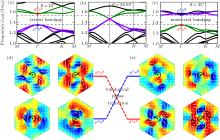Fig. 3.  (a)–(c) Band structures for the triangular phononic crystals with different rotational angles: (a) θ = 15°, (b) θ = 24.85°, and (c) θ = 35°. Green and violet dotted lines represent the bands including high-order compound modes pAsB and dAsB, respectively. (d), (e) Pressure field distributions of the modes pAsB and dAsB for phononic crystals shown in (a) and (c), respectively. The color patterns show the pressure distributions, where dark red and dark blue denote the positive and negative maxima, respectively. White arrows indicate the direction and amplitude of the time-averaged intensity. Black arrows show the spinning of sound intensity around the primitive cell center. The periodic boundary conditions are applied on the boundaries of the primitive cell.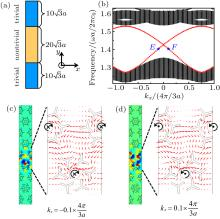Fig. 4.  (a) Schematic view of a ribbon-shaped supercell composed of the topologically nontrivial crystal (θ = 35°) with its two edges cladded by topologically trivial crystal (θ = 15°). (b) Projected band structure for the ribbon-shaped supercell along the Γ K direction. The black and red dotted lines denote bulk and edge states, respectively. (c), (d) Pressure field distributions confined around the interface between two domains of distinct topology at points E and F indicated in (b), respectively. The corresponding intensity is displayed in the magnified views. The color patterns show the pressure distributions, where dark red and dark blue denote the positive and negative maxima, respectively. Red arrows show the direction and amplitude of the time-averaged intensity. Black arrows show the spinning of sound intensity around the primitive cell center. The periodic boundary conditions are applied on the x and y directions of the ribbon-shaped supercell.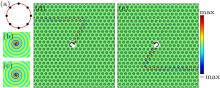Fig. 5.  (a) Schematic view of a point-like chiral source consisting of eight antennas, which has a phase difference of π/4 between the neighboring antennas. (b), (c) Pressure field distributions excited by the point-like chiral sources with clockwise and anticlockwise phase delays, respectively, in the air. (d), (e) Robustly unidirectional propagation of edge states along the Z-shaped interface between the topologically nontrivial and trivial crystals. The excitations in (d) and (e) correspond to the sources shown in (b) and (c), respectively. The white circle indicates the position of the point-like chiral source with operating frequency f ≈ 1.4027c0/a, whose phase delay direction is represented by the black arrow. The whole structure is surrounded by the perfectly matched layers to absorb outgoing waves.